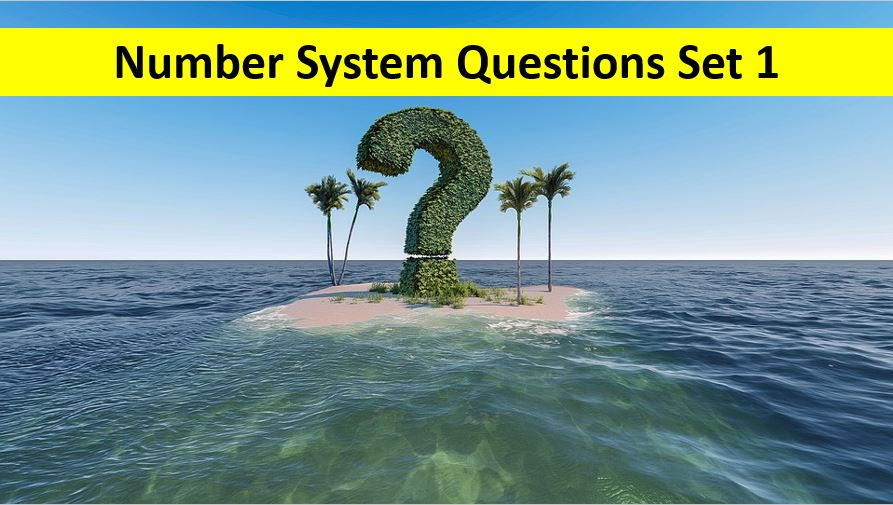Monday, September 27, 2021
Home > number system > Number System Question and Answer Set 1

# Number System Question and Answer Set 1# Number System Question and Answer Set 1

Hi students, welcome to Amans Maths Blogs (AMB). On this post, you will get the Number System Question and Answer Set 1 is the questions with solution for SSC CGL CHSL CAT and other competative exams like NTSE NSEJS NMTC etc. It will help you to practice the questions on the topics of maths as Number System.

Number System Questions and Answer Set 1: Ques No 1

The difference between two positive numbers is 3. If sum of their squares is 369, then the sum of the numbers is,

Options:

A. 25

B. 81

C. 27

D. 33

Number System Questions and Answer Set 1: Ques No 2

The sum of the factors of a number is 124. What is the number?

Options:

A. Number lies between 40 and 50

B. Number lies between 50 and 60

C. Number lies between 60 and 80

D. More than one such number exists

Number System Questions and Answer Set 1: Ques No 3

What least number must be added to 1056, so that the sum is completely divisible by 23?

Options:

A. 2

B. 3

C. 18

D. 21

Number System Questions and Answer Set 1: Ques No 4

Which of the following statements is INCORRECT?

Options:

A. If ‘a’ is a rational number and ‘b’ is irrational, then a + b is irrational.

B. The product of a non-zero rational number with an irrational number is always irrational.

C. Addition of any two irrational numbers can be rational.

D. Division of any two integers is an integer.

Number System Question and Answer Set 1: Ques No 5

N is a two-digit prime number. When the digits are interchanged, we get another prime number M. If N + M = 110, then find |N – M|

Options:

A. 18

B. 36

C. 54

D. 9

Number System Question and Answer Set 1: Ques No 6

Find the number of factors of 4200.

Options:

A. 48

B. 46

C. 56

D. 64

Number System Question and Answer Set 1: Ques No 7

Find the sum of divisors of 4200 (including 1 and 4200)

Options:

A. 560

B. 2880

C. 14880

D. 10679

Number System Question and Answer Set 1: Ques No 8

Find the product of all divisors of 84.

Options:

A. 12676

B. 21446

C. 36285

D. 844

Number System Question and Answer Set 1: Ques No 9

The number of ways in which 7056 can be expressed as the product of two positive integers is.

Options:

A. 12

B. 23

C. 36

D. 19

Number System Question and Answer Set 1: Ques No 10

The number of ways in which 12! can be expressed as the product of two positive integers is

Options:

A. 340

B. 396

C. 185

D. 198

# Number System Question and Answer Set 1 : Solutions

Let two positive numbers a and b. Then, a – b = 3 and a2 + b2 = 369.

Now, (a – b)2 = 9 ⇒ a2 + b2 – 2ab = 9 ⇒ 369 – 2ab = 9 ⇒ 2ab = 360,

So, (a + b)2 = a2 + b2 + 2ab = 369 + 360 = 729 ⇒ (a + b) = 27

Since the number N = paqbrc will have (a + 1) (b + 1)(c + 1) factors, where p, q, r are prime.

Thus, any number N of the form paqbrc, the sum of the factors will be (1 + p1 + p2 + p3 + …+ pa) (1 + q1 + q2 + q3 + …+ qb) (1 + r1 + r2 + r3 + …+ rc).

Sum of factors of number N = 124 = 22 * 31.

It can be written as 4 * 31, or 2 * 62 or 1 * 124.

Since 2 cannot be written as (1 + p1 + p2 + p3 + …+ pa) for any value of p, 4 can be written as (1 + 3) So, we need to see if 31 can be written in that form.

Now, 31 can be written in two different ways as 31 = (1 + 21 + 22 + 23 + 24) and 31 = ( 1 + 5 + 52)

Thus, the number N can be 3 * 24 or 3 * 52. Or N can be 48 or 75.

Since 1056 = (45 x 23) + 21, thus, to be divisible by 23, we need to add 23 – 21 = 2 to 1056.

Division of two integers may or may not be integer.

N = 10x + y, then M = 10y + x.

N + M = 11(x + y) ⇒ 110 = 11(x + y) ⇒ x + y = 10.

Thus, (x, y) = (3, 7) or (7, 3) as N = 10x + y = 37 or 73 both are prime numbers.

Therefore, |N – M| = |73 – 37| = 36

4200 = 23 x 31 x 52 x 71

Thus, Number of factors of 4200 = (3 + 1)(1 + 1)(2 + 2)(1 + 1) = 48

Since 4200 = 23 x 31 x 52 x 71, then the sum of divisors is

[(23+1 – 1)/(2-1)][(31+1 – 1)/(3-1)][(52+1 – 1)/(5-1)][(71+1 – 1)/(7-1)]

= 15 x 4 x 31 x 8 = 14880

Since 84 = 22 x 31 x 71, then the product of all divisors of 84 is

84(2+1)(1+1)(1+1)/2 = 846 = (12 x 7)6 = 126 x 76